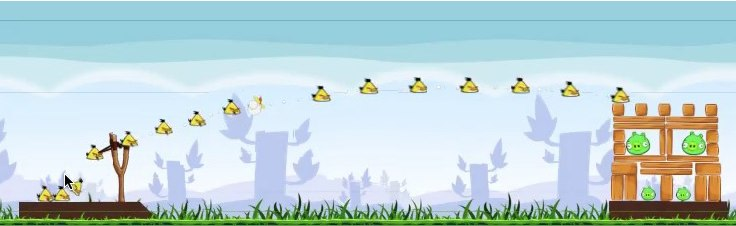# Projectile at two instants of timeA projectile thrown from ground moves at an angle of 30 degrees with the horizontal after 2 second of its flight and moves horizontally after further 1 second.

Calculate the initial velocity of the Projectile.

Note: Enter the answer as the $\left \lfloor v \right \rfloor$ where v is the answer to the problem Take $g = 10m/s^2$

×